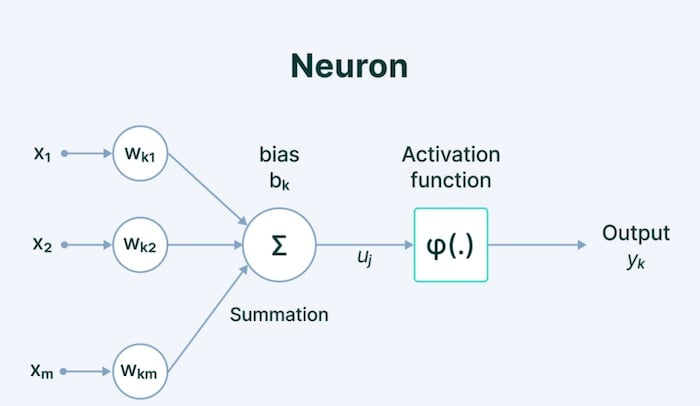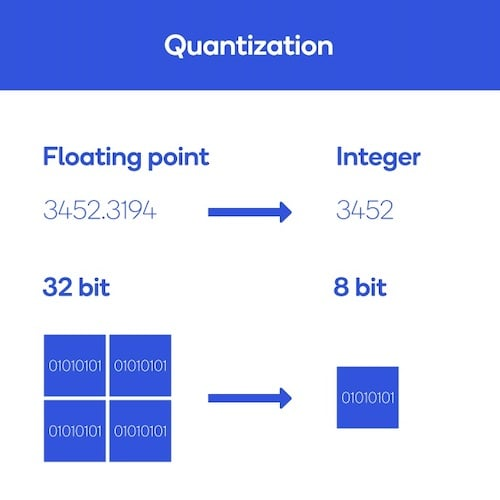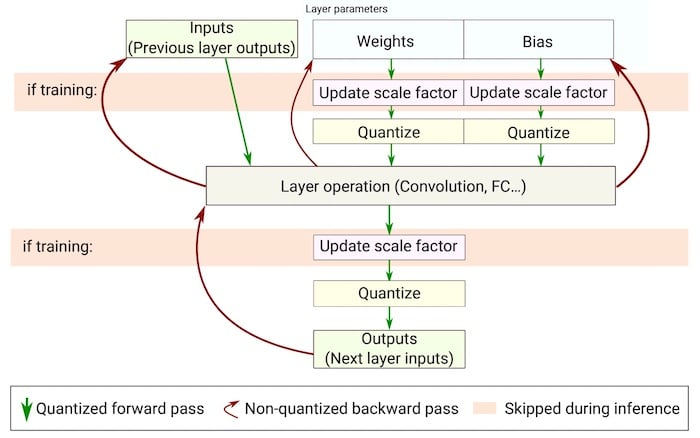Technical Article

# Neural Network Quantization: What Is It and How Does It Relate to TinyML?

March 06, 2022 by Jake Hertz

## This article will give a foundational understanding of quantization in the context of machine learning, specifically tiny machine learning (tinyML).

The primary challenge in tinyML is how to take a relatively large neural network, sometimes on the order of hundreds of megabytes, and make it fit and run on a resource-constrained microcontroller while maintaining a minimal power budget. To this end, the most effective technique is known as quantization.

This article will provide a foundational understanding of quantization, what it is, how it's used, and why it's important.

### Memory Constraints for Tiny Machine Learning Neural Networks

Before understanding quantization, it's important to discuss why neural networks, in general, take up so much memory

A neural network consists of a series of interconnected neurons in a series of layers. As shown in Figure 1, a standard neural network consists of layers of interconnected neurons, each with its own weight, bias, and activation function associated with it.##### Figure 1. An example of a neuron within a neural network. Each connection between neurons has its own weight, while each neuron has its own bias and activation function. Image used courtesy of V7 Labs

These weights and biases are often referred to as the "parameters" of a neural network.

Each neuron also has its own "activation," which is a number that dictates how active that neuron will be. The activation of a neuron is based on both its weight and bias values, as well as the activation function used.

The weights and biases are the parameters that then get tuned during training, and by extension, so does the neuron's activation.

These values, the weights, biases, and activations, are the majority of what gets physically stored in memory by a neural network. The standard is to represent these numbers as 32-bit floating-point values, which allows for a high level of precision and, ultimately, accuracy for the neural network.

This accuracy is why neural networks tend to take up so much memory. For a network with millions of parameters and activations, each being stored as a 32-bit value, memory usage quickly adds up.

For example, the 50-layer ResNet architecture contains roughly 26 million weights and 16 million activations. By using 32-bit floating-point values to represent both the weights and the activations, the entire architecture would require 168 MB of storage.

### What is Quantization for Neural Networks?

Quantization is the process of reducing the precision of the weights, biases, and activations such that they consume less memory

In other words, the process of quantization is the process of taking a neural network, which generally uses 32-bit floats to represent parameters, and instead converts it to use a smaller representation, like 8-bit integers.

Going from 32-bit to 8-bit, for example, would reduce the model size by a factor of 4, so one obvious benefit of quantization is a significant reduction in memory.

An example is shown in Figure 2.##### Figure 2. Quantization shrinks neural networks by decreasing the precision of weights, biases, and activations. Image used courtesy of Qualcomm

Another benefit of quantization is that it can lead to lower network latency and better power efficiency.

Network speed is improved because operations can be performed using integer, rather than floating-point data types. These integer operations require fewer computations on most processor cores, including microcontrollers.

Overall, power efficiency is then improved both because of decreased computation and decreased memory access.

Despite the benefits, the tradeoff with quantization is that neural networks can lose accuracy since they are not representing information precisely. However, depending on how much precision is lost, the network architecture, and the network training/quantization scheme, it has been shown that quantization can often result in a very minimal loss of accuracy, especially when weighed against the improvements in latency, memory usage, and power.

### How To Quantize a Machine Learning Model

In practice, there are two main ways to go about quantization:

As the name implies, post-training quantization is a technique in which the neural network is entirely trained using floating-point computation and then gets quantized afterward.

To do this, once the training is over, the neural network is frozen, meaning its parameters can no longer be updated, and then parameters get quantized. The quantized model is what ultimately gets deployed and used to perform inference without any changes to the post-training parameters.

Though this method is simple, it can lead to higher accuracy loss because all of the quantization-related errors occur after training is completed, and thus cannot be compensated for.

Quantization-aware training, as shown in Figure 3, works to compensate for the quantization-related errors by training the neural network using the quantized version in the forward pass during training.##### Figure 3. Flow chart of quantization-aware training. Image used courtesy of Novac et al

The idea is that the quantization-related errors will accumulate in the total loss of the model during training, and the training optimizer will work to adjust parameters accordingly and reduce error overall.

Quantization-aware training has the benefit of much lower loss than post-training quantization.

For a much more in-depth, technical discussion about the mathematics behind quantization, it is recommended to read this paper from Gholami, et al.

### Quantization for TinyML

For TinyML, quantization is an invaluable tool that is at the heart of the whole field.

All in all, quantization is necessary for three main reasons:

1. Quantization significantly reduces model size—this makes it more feasible to run ML on a memory-constrained device like a microcontroller.
2. Quantization allows for ML models to run while requiring less processing capabilities—MCUs used in TinyML tend to have less performant processing units than a standard CPU or GPU.
3. Quantization allows for a reduction in power consumption—the original goal of TinyML was to perform ML tasks at a power budget under 1mW. This is necessary to deploy ML on devices powered by small batteries like a coin cell.